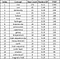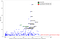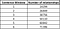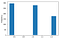# Automated generation of a concept map from text

In my previous post, concepts were extracted from an electronic textbook. The next step is to simulate an expert’s mind map based on the concepts extracted. For that we would need some way of computing which concepts are important, which concepts are related, how they’re related, and how important those relationships themselves are. I’m using a wikipedia article on stars for this exercise. The text was obtained with BeautifulSoup.

# Concept Importance:

Ranking the concepts by their importance shows the student on which concepts to focus their efforts. In the absence of annotated data on which concepts are important for learning about stars, let’s evaluate the following hypotheses:

Hypothesis 1 — Raw counts: The more times a concept is mentioned in the text, the more important it is. Above is the distribution of raw counts of concepts in the text. Almost 1200 of the concepts extracted from the wikipedia article are mentioned<10 times. Not visible is the concept phrase ‘star’ (174 counts).

Zooming in further on raw counts in the image below shows a few concepts (~5) with raw counts above 20.

It should be noted that several concept phrases have already been removed based on a list of generic phrases such as ‘image’ (2 counts), ‘function’ (4 counts), ‘type’ (13 counts), ‘example’ (10 counts), group (10 counts), set (2 counts), technique (2 counts), term (5 counts) system (30 counts). These phrases are used frequently but do not add much meaning. Since this list of ‘stop concepts’ is not exhaustive, raw counts may prune some unimportant concepts, but includes other generic concepts such as ‘time’ (25 counts) and ‘year’ (17 counts).

Hypothesis 2 — TFIDF: If the concept is uncommon outside of this text, but frequent in this text, it is more important. (The Term Frequency-Inverse Document Frequency ratio, or TFIDF). The Inverse Document Frequency (IDF) is computed with a large collection of documents (corpus), typically in a similar subject area or genre as the text being analyzed. Assuming a typical adult readability for the documents, the Reuters corpus available from the NLTK python library was chosen. It contains 10,788 news articles and around 1.3 million words. Computing the IDF values for two word or three word concept terms was getting computationally expensive, however, so as a gross approximation, the highest IDF value of the individual words was used, with a multiplier based on the number of terms contained in the concept phrase. Below is a table of concepts ranked by TF-IDF.Concepts ranked by TF-IDF

In spite of a low IDF value, star has a high enough raw count of mentions to make it the most important concept. The next few concepts are sun, luminosity, core and helium. Concepts ‘helium’, ‘astronomer’, and ’supernova’ were bumped up above lower raw count concepts because of having a high IDF value — mentioned rarely in the NLTK Reuters corpus. High raw count concepts ‘year’ and ‘time’ were much lower in this ranking and not tagged as particularly important. This seems to be a reasonable way to order concept importance, although a bigger corpus in the general subject area of the analyzed text would definitely perform better.

Hypothesis 3 — Spread: If the concept is encountered throughout the text, it is more important than if it is only mentioned in one part of the text. A concept may have high raw count but low spread if it is only important in one subsection of the text as opposed to throughout the text. To compute spread, a numeric index was assigned to the sentences in the text. The spread was calculated as the standard deviation of the sentences that contained the concept phrase, normalized to the total length of the text in sentences (423 total sentences). Not visible is the concept ‘star’ with a spread of 0.28.

The spread may be large if an unimportant concept is mentioned three times but spread out in the text (‘stellar atmosphere’ and ‘gravitational collapse’, 2 counts each). Spread computed by sentence indices could also be affected by the ordering of subsections of text dealing with related content. So spread could be an artefact of the writing style and length of text, and is not a useful indicator by itself.Raw counts vs Spread

The figure below looks at the spread of the high TFIDF concepts shows which concepts are important in a subsection of the text vs important all around the text. The concepts ‘rotation’ and ‘magnetic field’ have a similar raw count to ‘radius’ and ‘white dwarf’, but are important in fewer contexts (in this particular text). Similarly, ‘massive star’ is mentioned in fewer contexts than ‘earth’, although ‘earth’ has a similar raw count. Focusing on learning about high TFIDF, high spread concepts first will help get the most out of the text.

# Relationship Strength:

Based on the assumption that the proximity of two concepts indicates relatedness, a preliminary graph was built. If the concepts were mentioned within a window of a number of sentences, they were considered related. To avoid an extremely dense map (capturing unimportant or distant relationships) or a map that is too sparse (relationships not captured), as a first guess, the window was set to 1 sentence before and after — if a concept y is in two sentences before or after concept x, then concept x and concept y were considered related. It should be noted that pronouns have not been included in the analysis.Number of relationships captured by varying sentence window

How strongly related are two concepts? What are the most important connections a concept has? Three heuristic metrics were computed and compared:

## Metric 1: Sentence distance count

Assuming that if two concepts are mentioned in the same sentence, they are strongly related, my first guesses were that the relationship strength would be directly proportional to how often they occur closeby, and indirectly proportional to the distance between them. Accordingly, a preliminary heuristic metric for each pair of concepts x and y was calculated as:

where sentence distance s is the difference of how many sentences separate the two concepts, starting from the same sentence (s = 0) to s = S, where S is the maximum distance at which concepts are considered related (typically set between 0 and 3).

To calculate relationship strength relative to one of the concepts, the average Sentence distance count of concept x with every related concept is subtracted from the Sentence distance count of concept x to concept y

A quick look at the sorted concepts shows the limitations of this metric.

1. For relatively smaller articles, with a reasonable threshold sentence distance (S= 3), this metric is not granular enough, showing over 150 concepts with the same high relationship strength. This does not help us meet our objective of screening which relationships are most important to focus on first.Sentence distance metric

2. Infrequently mentioned concepts are unfairly rewarded for being mentioned in the same sentence once. This would mean that if two concepts are mentioned once in the same sentence, the relationship is as important as if the concepts are mentioned twice with a sentence distance = 2, or if the two concepts are mentioned three times with a sentence distance = 3.

3. This metric does not help us understand for which concept the relationship is important. Is ‘nuclear fuel’ an important concept to ‘star’, or is it the other way around?

## Metric 2: Common concept Jaccard similarity coefficient

How many concepts are both concept x and concept y related to? A Jaccard similarity metric for common concepts can be computed as:

where xmap is the set of all concepts x is related to, and ymap is the set of all concepts to which y is related. The Jaccard similarity coefficient(cardinality of intersection divided by cardinality of union) of these two sets may provide a measure of how important the relationship is.

This metric has similar limitations to metric 1. A long sentence containing a lot of concept terms will skew this number. The following sentence from the wiki page illustrates this:

`In the Sun, with a 10-million-kelvin core, hydrogen fuses to form helium in the proton–proton chain reaction: These reactions result in the overall reaction: where e+ is a positron, γ is a gamma ray photon, νe is a neutrino, and H and He are isotopes of hydrogen and helium, respectively.`

Although concepts ‘positron’ and ‘chain reaction’ are only mentioned once, a longer sentence skews the results.

## Metric 3: Co-occurence

Another heuristic metric defined to capture how closely two concepts co-occur was computed.

For every mention of concept x in the text, the distance to the nearest mention of concept y was found. The median of these shortest distances provides a measure of how closely the concept y is related to concept x. If the value is relatively small, then for most mentions of concept x, there is a concept y nearby. This would make concept y an important relationship for concept x.

This metric is a lot more granular, and since the value for concept x to concept y can be different from the value for concept y to concept x, it indicates the relative importance of the relationship for each concept.

From the above table, we see that 50% of the mentions of ‘star’ are less than or equal to 29 sentences away from a mention of ‘light-year’, while 50% of the mentions of ‘light-year’ are less than a sentence away from the nearest mention of ‘star’. So ‘star’ is an important relationship for ‘light-year’. The concept ‘space’ is somewhat more important to ‘energy’ than the other way around.

In the absence of a multi-sourced annotated dataset on the strength of relationships, we have to make some assumptions. If we posit that language develops vocabulary to represent strong relationships succinctly, then the first metric (Sentence distance count) has merit. The relationship may be strong but not important in the context of the text being analyzed.

The second and third metrics actually answer a slightly different question — how important the relationship is in this text. In other words, while reading and comprehending the text, how often will both concepts occupy space in the reader’s working memory? The common concept Jaccard similarity index was found to skew heavily with complex sentences, unfairly rewarding infrequent concepts that were mentioned along with many others. The co-occurrence metric does not have the same limitation. It also varies with respect to concept, as the relationship may be much more important to one of the concepts.

If we restrict the concepts to the top 5% of TFIDF values and top 5% of spread (measures of concept importance), and the top 1% of co-occurring concepts (measure of relationship importance), the below partial graph is generated.

The first step to learning about stars in the article would be to understand the concepts in the above graph, and their relationship to ‘star’. The above analysis provides a good starting point for learning about any concept in a text. It becomes especially useful when the amount of text is vast.

# Next steps

Now that we know which concepts are important, and which relationships are important to a concept, we will look into extracting the type of relationships being extracted. This will enable us to store what we learn in a knowledge base, and for the intelligent tutoring system to ask smart questions.

We can also start constructing personalized learning paths. In the next articles, we will deal with building a personalized learning path. Setting learning objectives and figuring out what you already know is crucial while navigating a massive amount of text. The learning path will be based on individual student’s prior knowledge, and will simulate their cognitive load so the student is not bored (repeating known concepts) or overwhelmed (too many new concepts introduced at once).